Latest Banking jobs   »   Reasoning Ability Quiz For IBPS RRB...

# Reasoning Ability Quiz For IBPS RRB PO Clerk Prelims 2022- 24th July

Directions (1-5): Study the information carefully and answer the questions given below.
Eight persons sit around a rectangular table such that two persons sit on each side of the table. The persons sitting on the shorter side of the table face away from the center and the persons sitting on the longer side of the table face toward the center. The persons having names with consecutive letters according to English alphabet do not sit opposite to each other.
E is one of the persons. A is immediate left to G who sits at the shorter side of the table. H is 2nd right to F. C is immediate left to B who sits opposite to D. A sits opposite to one of the immediate neighbor of C.

Q1. Who among the following sits opposite to H?
(a) E
(b) G
(c) C
(d) B
(e) none of these

Q2. Who among the following is 4th to the left of the one who is 2nd left to A?
(a) E
(b) F
(c) C
(d) B
(e) none of these

Q3. Which of the following represents the position of G with respect to C?
(a) Immediate left
(b) Immediate right
(c) 2nd to the right
(d) 4th to the left
(e) None of these

Q4. Which of the following statement is true regarding B?
(a) E faces B
(b) F sits 3rd left to B
(c) B and G sit on the same side of the table
(d) B faces towards the center of the table
(e) All are true

Q5. How many persons sit between D and H, when counted from the left to H?
(a) Two
(b) One
(c) Three
(d) Four
(e) None of these

Direction (6-10): In each of the questions below, some statements are given followed by some conclusions. You have to take the given statements to be true even if they seem to be at variance with commonly known facts. Read all the conclusions and then decide which of the given conclusions logically follows from the given statements disregarding commonly known facts.
Q6. Statements: Only a few states is country.
Some country is city.
All city is village
Conclusions: I. All country can be city
II. All states can be country

(a) If only conclusion I follows.
(b) If only conclusion II follows.
(c) If either conclusion I or II follows
(d) If neither conclusion I nor II follows.
(e) If both conclusions I and II follow.

Q7. Statements: Some Sania is Sindhu
No Sindhu is Player
All Player is India
Conclusions: I. Some Sindhu can be India
II. Some Sania is not player

(a) If only conclusion I follows.
(b) If only conclusion II follows.
(c) If either conclusion I or II follows
(d) If neither conclusion I nor II follows.
(e) If both conclusions I and II follow.

Q8. Statements: Only a few states is country.
Some country is city.
All city is village
Conclusions: I. All country can be states
II. Some village can be country

(a) If only conclusion I follows.
(b) If only conclusion II follows.
(c) If either conclusion I or II follows
(d) If neither conclusion I nor II follows.
(e) If both conclusions I and II follow.

Q9. Statements: Only flower is fruit
Some flower is chocolate
Some chocolate is drink
Conclusions: I. Some fruit can be drink
II. Some flower can be drink

(a) If only conclusion I follows.
(b) If only conclusion II follows.
(c) If either conclusion I or II follows
(d) If neither conclusion I nor II follows.
(e) If both conclusions I and II follow.

Q10. Statements: All flower is fruit
All fruit is chocolate
No chocolate is drink
Conclusions: I. Some flower can be chocolate
II. Some fruit can be drink

(a) If only conclusion I follows.
(b) If only conclusion II follows.
(c) If either conclusion I or II follows
(d) If neither conclusion I nor II follows.
(e) If both conclusions I and II follow.

Q11. There are five persons i.e., P, Q, R, S and T. If S is shorter than T and P. Q is taller than T who is not the taller than P. Q is taller than only two persons. P is not the tallest, then who is the second tallest person among all?
(a) P
(b) S
(c) Q
(d) R
(e) None of these

Directions (12-13): Study the information carefully and answer the questions given below.
J, K, L, M, N and O are six members of a family. There are equal numbers of male and female in the family. J is the son of M and N is the daughter of J. K is the child of L who is the mother of N. O is paternal grandmother of K.

Q12.How is O related to L?
(a) Daughter-in-law
(b) Mother-in-law
(c) Mother
(d) Aunt
(e) None of these

Q13.How is K related to M?
(a) Daughter
(b) Father
(c) Son
(d) Grandson
(e) Granddaughter

Directions (14-15): Study the following information carefully and answer the given questions.
Amongst Six friends A, B, C, D, E and F, each got different marks in the examination. E score more than A. C score less than F. More than three persons score less than B. E does not score more than C. A does not score lowest marks and F does not score highest marks. The one who scores second lowest score 60 marks.

Q14. Who among the following person score fourth highest?
(a) C
(b) A
(c) E
(d) D
(e) None of these

Q15. If C score 80 marks, then what may be the score of E?
(a) 63 marks
(b) 70 marks
(c) 52 marks
(d) Either (a) or (b)
(e) Can’t be determined

Solutions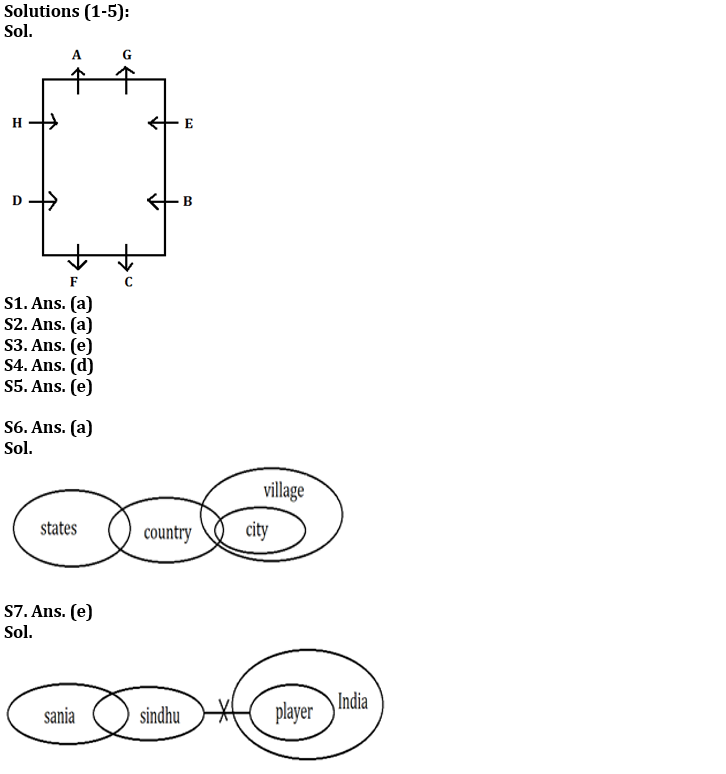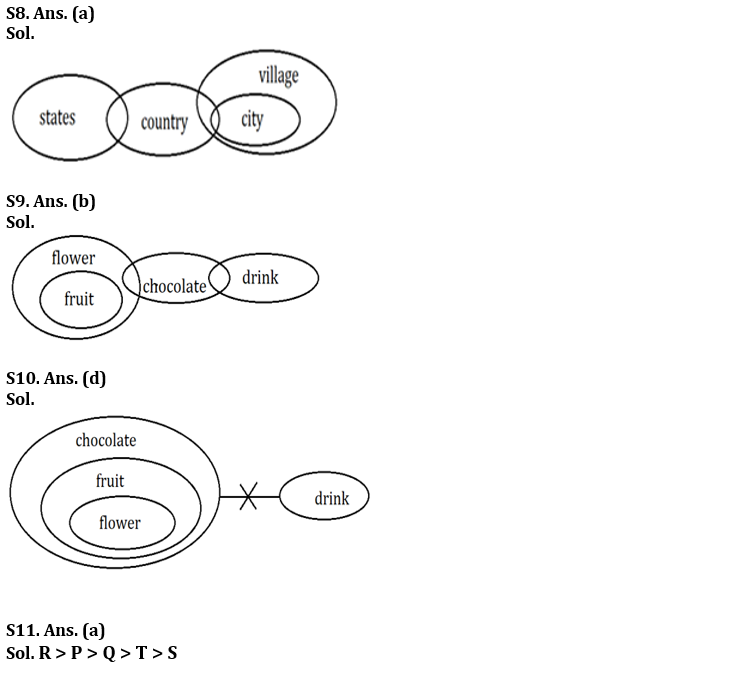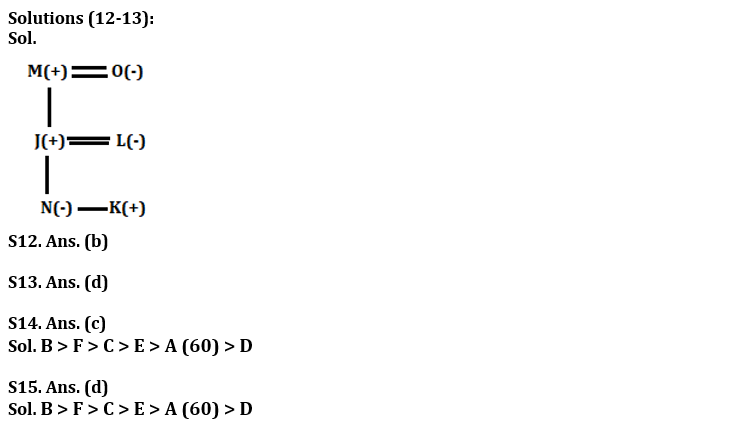#### Congratulations!Incorrect details? Fill the form again here

•Reasoning Ability Quiz For IBPS RRB PO C...
•Quantitative Aptitude Quiz For IBPS RRB ...
•Reasoning Ability Quiz For IBPS RRB PO C...
•Quantitative Aptitude Quiz For IBPS RRB ...
•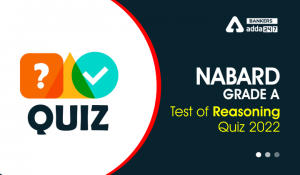Reasoning Ability Quiz For NABARD Grade ...
•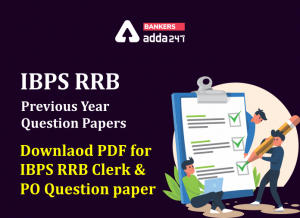IBPS RRB Previous Year Question Paper PD...